Data analysis using mixed models with ASReml

HomeBlogs# Data analysis using mixed models with ASReml

Dr. Arthur Gilmour

24 May 2022ASReml caters for a wide range of linear mixed models. Some models are quite complex involving hundreds of diverse variance components, each model has its own characteristics and some involve special ‘tricks’ in their specification. In addition, some datasets are quite large with millions of fixed and random effects, requiring the use of efficient computational tools, such as those exploiting sparsity. In the following sections, I describe some of these challenges and provide some details to consider.

### Processing time

Gilmour (2019, DOI: 10.1111/jbg.12398) describes the process of fitting linear mixed models as implemented in ASReml. That paper highlights the principle of minimising the computation by the use of sparsity, an important requirement for fast processing. A second requirement is the efficiency of numerical calculations and this has been significantly enhanced in ASReml 4.2 (build ng, May 2022) in particular by rearranging the computation. Consequently, this build of stand-alone ASReml is up to 100 times faster than ASReml 4.1 (available in 2019).

### An appropriate model

There are some widely used, well founded mixed models such as the animal model, the sire model, maternal model, spatial analysis as well as basic regression, factorial and (in)complete block analyses. These become the building blocks for multi-trait and multi-environment analyses. Each data set has its own characteristics and these should always be recognised. For example, litters in extensively grazed sheep are not biologically equivalent to litters in intensively housed pigs! Potential issues include the distribution of the data (binary, normal, skewed), variance heterogeneity, outliers and missing values. In addition, there is the issue of the purpose of the analysis: to select individuals for breeding, test a treatment hypothesis, estimate heritability, understand genetic and phenotypic correlations. All of these affect the type and structure of the model fitted.

### Incremental complexity

It is important to perform univariate/single site analyses first before considering multi-variate/multi-environment analyses since the latter will fail to estimate covariance if the former fail to establish there is variance. In a recent case a user wanted to fit a trivariate animal model with repeated records. The trivariate analysis failed. The model involved additive genetic effects (animal effects estimated with a numerator relationship matrix), permanent environment (PE) effects (uncorrelated animal effects), fixed contemporary group effects and correlated residuals. However, for the second trait, there was no PE variance and for the third trait, there were no repeat observations although the data was set up with the animal effect repeated. Consequently, with no ‘residual’ (sampling) variance, univariate analysis of the third trait failed. After modifying the data file so that the third trait only appeared once for each animal, the trivariate analysis was performed with 3 variances and covariances at the genetic level, 2 variances and a covariance at the animal level (1 and 3) and 2 variances and a covariance at the sampling level (1 and 2).

### Big Data

Many datasets are quite big and consequently models become quite large. The issue then becomes one of sparsity. With field trials, a spatial analysis based on an autoregressive correlation of plots within rows and within columns has become common because it accommodates most field correlation patterns well and is sparse in that only immediate neighbours are connected in the inverse residual variance matrix. Similarly, very large data sets can be analysed using the numerator relationship matrix to define genetic links because the inverse is sparse with links between parents and their offspring and between parents with common offspring. This sparsity is exploited successfully by ASReml.

Similarly, if we want a covariance matrix across many traits/environments, it is likely to be over parameterised. The Factor Analytic variance structure allows the matrix to be estimated with reduced parameterization (in the spirit of principal components) and has been formulated to fit more sparsely as loadings plus specific variances. This formulation also helps avoid getting negative definite covariance matrices.

### Processing efficiency

In specifying a model in ASReml, the terms are organised in 3 groups.  The first group is for small fixed factors for which the order of fitting is important because of the desire to perform significance testing using Wald F statistics. The equations for these are likely to be relatively dense, especially after adjusting for the other terms in the model, but there are typically less than 1000 of them. The second group is for random factors; a variance structure is declared for each. The third group is for large fixed effects (no variance structure). After forming the mixed model equations, ASReml reorders the equations in the last 2 groups to retain as much sparsity as possible. More sparsity means less computation (fewer elements of the sparse inverse to compute). Generally, this reordering has no impact on the answers (effects estimated) unless the fixed model is over-parameterised in which case different equations may be declared singular.

More recent developments in ASReml include storing and processing the sparse matrices more efficiently and changing the order of computation to reduce reading and writing to memory. It can also successfully access more RAM, allowing even larger models to be fitted.

### User support

ASReml is designed to cater for a wide range of linear mixed models, being extensively used in plant and animal breeding, forestry and for analysis of human epidemiological data. That breadth of coverage is sometimes daunting to new users as most options are only relevant to particular situations. VSNi is committed to helping new and experienced users identify the most appropriate model for their data although VSNi does not provide an analysis service as such; but the developers of ASReml have broad experience as biometricians/statisticians.

### Final words

The linear mixed model, as implemented in ASReml, has allowed immense advances in global food productivity and health (plant, animal and human) via quantitative and marker-based genetics over the last 25 years.

Arthur Gilmour obtained his BSc Agr from Sydney University majoring in Biometry in 1970 with a NSW Government traineeship. He served as a biometrician until his retirement from NSW Agriculture as a Principal Research Scientist in 2009. The generalized linear mixed model has been the major research interest of Dr Gilmour, motivated by problems arising in research data generated by agricultural scientists. From the outset, he was involved in software development to meet the current statistical analysis needs of his clients and colleagues. He obtained his PhD in animal breeding from Massey University in 1983 during which time he came into contact with Robin Thompson. This led to an ongoing collaboration also with Brian Cullis, resulting in the development of ASReml in 1996.

02 March 2022

Significance levels: a love-hate relationship

We frequently hear in the scientific community a debate in favour of or against the use of p-values for statistical testing. The focus of this note is not to contribute (or muddle) this debate, but just to bring attention to a couple of elements critical to understanding the concept of the significance level. We will focus on three keywords: convention, trade-off and risk.

Recall that significance level refers to that value, say α = 0.05, that we use to decide if we should reject a null hypothesis (e.g., no difference in the means between two treatments). This α value represents the proportion of mistakes (here 5%) that we will make when we reject the null hypothesis when in fact it is true. This type I error, implies that we declare two means significantly different when in fact they are not (an undesirable situation with a false positive). Recall that we also have type II error, or β, the case in which we do not declare two means significantly different when in fact they are (another undesirable situation). This last error is associated with the power of a test, but here we will only focus on the controversial α value.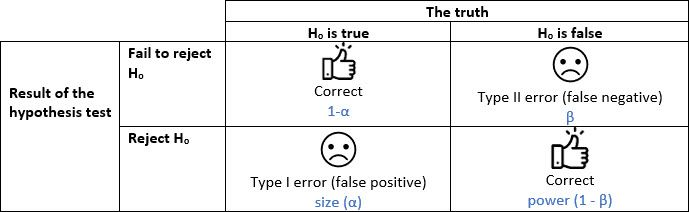Let’s start with the keyword convention. Depending on the field, we use different significance levels to ‘dictate’ when a test result is significant. Often, we use 0.10, 0.05 or 0.01; the use of a higher value usually is associated with scientific fields where there are greater levels of uncertainty (e.g., ecology), whereas stricter (lower) values are used in medical or pharmaceutical studies.

Without a doubt, the most common value used is 0.05: but why this value and what does it mean? This value, as indicated earlier, indicates that if the null hypothesis is true, we will only reject it wrongly 5% of the time (or 1 in 20 times). This seems a reasonable number, but a somehow arbitrary choice. This significance level originated from the tables of critical values reported by Fisher and Yates back in 1938 and this value has stayed as a convenient number. (However, they also reported 0.10, 0.02, 0.01, 0.002 and 0.001). In part these significance levels were chosen by the above authors given their limitations to calculate all possible probabilities. Now, we usually get p-values instead of critical values, but interestingly, we still focus on that historical reference of 5%.

The convenient and arbitrary choice of a 5% significance level has now become a convention. But there is no scientific or empirical reasoning for the use of this or any other value. This is one of the reasons for the present debate on its use. It also seems odd that, for example, when α is 0.05 we declare a test significant with a p-value of 0.047, but not significant if this p-value is 0.053. As you can see this enhances its arbitrary nature, and maybe we should focus on the strength of the evidence against our null hypothesis, namely, talking about mild or strong significance in our results, instead of a yes/no attitude.

The second keyword is trade-off. In any decision, even in the simplest life decisions, there is always the possibility of making some mistakes. If, for your statistical inference, you are unwilling to make almost any mistakes, then you should select a very small value: an α, say of 0.0001 (here, 1 in 10,000 times you will make the wrong decision). This, at first glance, seems reasonable, but it has other implications. Requiring a very high level of confidence will mean that you will almost never reject the null hypothesis even if rejecting it is the correct decision! This occurs because you are setting up very strict thresholds to report a significant result and therefore you need extremely strong evidence.

The above conservative philosophy results in two side effects. First, a waste of resources as you are extremely unlikely to report significant differences from almost any study you might execute; only those with extremely large effects (or sample sizes) will have sufficient power. Second, the scientific advancement in terms of discoveries (for example, a new drug or treatment) will be hampered by these strict values. For example, we will have fewer drugs or treatments available for some illnesses. Here, it is the society as a whole that loses in progress and scientific advancement with such a high threshold.

On the other hand, something different, but also concernin, occurs with a very flexible significance level (say α = 0.30). Here we are very likely to find a ‘better’ drug or treatment, but these are not necessarily true improvements as we are likely having too many false positives with results almost random. This too has strong side effects for all of us such as: 1) a large societal cost on having drugs or treatments that are not necessarily better than the original ones (even if we think they are), and 2) it will be hard, as an individual, to discriminate between the good and the bad drugs (or treatments) as there are too many ‘good’ options available and all are reported as the ‘best’ ones!

This is where there exists a trade-off between too much or too little; individuals and societies need to define what is an adequate level of mistakes. Somehow it seems the scientific community has chosen 0.05! But any value will be always good or bad depending on the above trade-offs.

The last keyword is risk. Any decision that involves a mistake implies a risk. This is better understood with a few examples. So, imagine that you are considering using an alternative drug to treat your chronic disease that potentially has some bad, but manageable, side effects. In this case, you might want stronger evidence that this drug is going to be better than the standard; hence, you might want to use an α of 0.0001, to make sure it is better (at least statistically). Hence, under potentially high personal risk of a bad decision, the significance level required has to be lower.

In contrast, imagine that you want to use the seeds of an alternative tomato variety that has been recommended to you for planting in your garden. The risk of having the wrong genetic source is ever present, and it will imply a waste of resources (money, time and frustration); but, under failure, your risk is relatively low, as you can buy tomatoes from the supermarket and the following year, go back to the seeds of your typical (and well tested) variety. In this case, the personal risk is relatively low, and you might be happy with an α of 0.10 (implying 1 in 10 times you will be incorrect) to give a chance to this alternative variety.

Hence, it is difficult to define what is an adequate level of risk for our decisions, an aspect that is also subjective. And once more, it seems that the scientific community has decided that 5% is a good level of risk!

In summary, significance levels are a convention that we have carried for a while but going forward we need to be flexible and critical on how they are used and reported. In many senses they are subjective decisions, and we could even say they are individual decisions that take into consideration our personal like or dislike of risks and the potential trade-off in taking the right or wrong decisions. So, next time you see a p-value take a step back and think about the consequences!

Salvador Gezan is a statistician/quantitative geneticist with more than 20 years’ experience in breeding, statistical analysis and genetic improvement consulting. He currently works as a Statistical Consultant at VSN International, UK. Dr. Gezan started his career at Rothamsted Research as a biometrician, where he worked with Genstat and ASReml statistical software. Over the last 15 years he has taught ASReml workshops for companies and university researchers around the world.

Dr. Gezan has worked on agronomy, aquaculture, forestry, entomology, medical, biological modelling, and with many commercial breeding programs, applying traditional and molecular statistical tools. His research has led to more than 100 peer reviewed publications, and he is one of the co-authors of the textbook “Statistical Methods in Biology: Design and Analysis of Experiments and Regression”.

09 March 2022

Meta analysis using linear mixed models

Meta-analysis is a statistical tool that allows us to combine information from related, but independent studies, that all have as an objective to estimate or compare the same effects from contrasting treatments. Meta-analysis is widely used in many research areas where an extensive literature review is performed to identify studies that had a similar research question. These are later combined using meta-analysis to estimate a single combined effect. Meta-analyses are commonly used to answer healthcare and medical questions, where they are widely accepted, but they also are used in many other scientific fields.

By combining several sources of information, meta-analyses have the advantage of greater statistical power, therefore increasing our chance of detecting a significant difference. They also allow us to assess the variability between studies, and help us to understand potential differences between the outcomes of the original studies.

The underlying premise in meta-analysis is that we are collecting information from a group of, say n, studies that individually estimated a parameter of interest, say . It is reasonable to consider that this parameter has some statistical properties. Mainly we assume that it belongs to a Normal distribution with unknown mean and variance. Hence, mathematically we say:

In meta-analysis, the target population parameter θ can correspond to any of several statistics, such as a treatment mean, a difference between treatments; or more commonly in clinical trials, the log-odds ratio or relative risk.

There are two models that are commonly used to perform meta-analyses: the fixed-effect model and the random-effects model. For the fixed-effect model, it is assumed that there is only a single unique true effect our single θ above, which is estimated from a random sample of studies. That is, the fixed-effect model assumes that there is a single population effect, and the deviations obtained from the different studies are only due to sampling error or random noise. The linear model (LM) used to describe this process can be written as:

where is each individual observed response, is the population parameter (also often known as  μ, the overall mean), and is a random error or residual with assumptions of . The variance component is a measurement of our uncertainty in the information (i.e., response) of each study. The above model can be easily fitted under any typical LM routine, such as R, SAS, GenStat and ASReml.

For the random-effects model we still assume that there is a common true effect between studies, but in addition, we allow this effect to vary between studies. Variation between these effects is a reasonable assumption as no two studies are identical, differing in many aspects; for example, different demographics in the data, slightly differing measurement protocols, etc. Because, we have a random sample of studies, then we have a random sample of effects, and therefore, we define a linear mixed model (LMM) using the following expression:

where, as before, is each individual observed response, is the study-specific population parameter, with the assumption of and is a random error or residual with the same normality assumptions as before. Alternatively, the above LMM can be written as:

where and is a random deviation from the overall effect mean θ with assumptions .

This is a LMM because we have, besides the residual, an additional random component that has a variance component associated to it, that is or . This variance is a measurement of the variability ‘between’ studies, and it will reflect the level of uncertainty of observing a specific  . These LMMs can be fitted, and variance components estimated, under many linear mixed model routines, such as nlme in R, proc mixed in SAS, Genstat or ASReml.

Both fixed-effect and random-effects models are often estimated using summary information, instead of the raw data collected from the original study. This summary information corresponds to estimated mean effects together with their variances (or standard deviations) and the number of samples or experimental units considered per treatment. Since the different studies provide different amounts of information, weights should be used when fitting LM or LMM to summary information in a meta-analysis, similar to weighted linear regression. In meta-analysis, each study has a different level of importance, due to, for example, differing number of experimental units, slightly different methodologies, or different underlying variability due to inherent differences between the studies. The use of weights allows us to control the influence of each observation in the meta-analysis resulting in more accurate final estimates.

Different statistical software will manage these weights slightly differently, but most packages will consider the following general expression of weights:

where is the weight and is the variance of the observation. For example, if the response corresponds to an estimated treatment mean, then its variance is , with MSE being the mean square error reported for the given study, and the number of experimental units (or replication).

Therefore, after we collect the summary data, we fit our linear or linear mixed model with weights and request from its output an estimation of its parameters and their standard errors. This will allow us to make inference, and construct, for example, a 95% confidence interval around an estimate to evaluate if this parameter/effect is significantly different from zero. This will be demonstrated in the example below.

### Motivating example

The dataset we will use to illustrate meta-analyses was presented and previously analysed by Normand (1999). The dataset contains infromation from nine independent studies where the length of hospitalisation (measured in days) was recorded for stroke patients under two different treatment regimes. The main objective was to evaluate if specialist inpatient stroke care (sc) resulted in shorter stays when compared to the conventional non-specialist (or routine management) care (rm).

The complete dataset is presented below, and it can also be found in the file STROKE.txt. In this table, the columns present for each study are the sample size (n.sc and n.rm), their estimated mean value (mean.sc and mean.rm) together with their standard deviation (sd.sc and sd.rm) for both the specialist care and routine management care, respectively.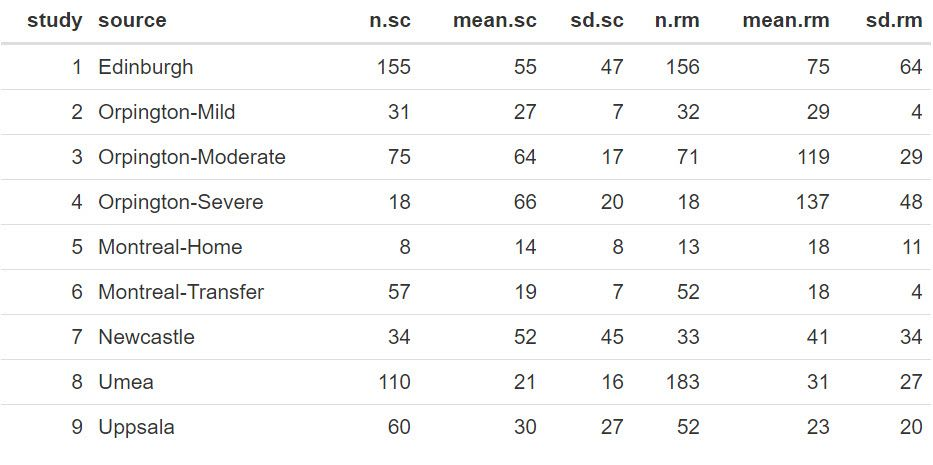### Statistical analyses

We will use the statistical package R to read and manipulate the data, and then the library ASReml-R (Butler et al. 2017) to fit the models.
First, we read the data in R and make some additional calculations, as shown in the code below:

 STROKE <- read.table("STROKE.TXT", header=TRUE)
 STROKE$diff <- STROKE$mean.sc - STROKE$mean.rm STROKE$Vdiff <- (STROKE$sd.sc^2/STROKE$n.sc) + (STROKE$sd.rm^2/STROKE$n.rm) STROKE$WT <- 1/(STROKE$Vdiff)

The new column diff contains the difference between treatment means (as reported from each study). We have estimated the variance of this mean difference, Vdiff, by taking from each treatment its individual MSE (mean square error) and dividing it by the sample size, and then summing the terms of both treatments. This estimate assumes, that for a given study, the samples from both treatments are independent, and for this reason we did not include a covariance. Finally, we have calculated a weight (WT) for each study as the inverse of the variance of the mean difference (i.e., 1/Vdiff).

We can take another look at this data with these extra columns: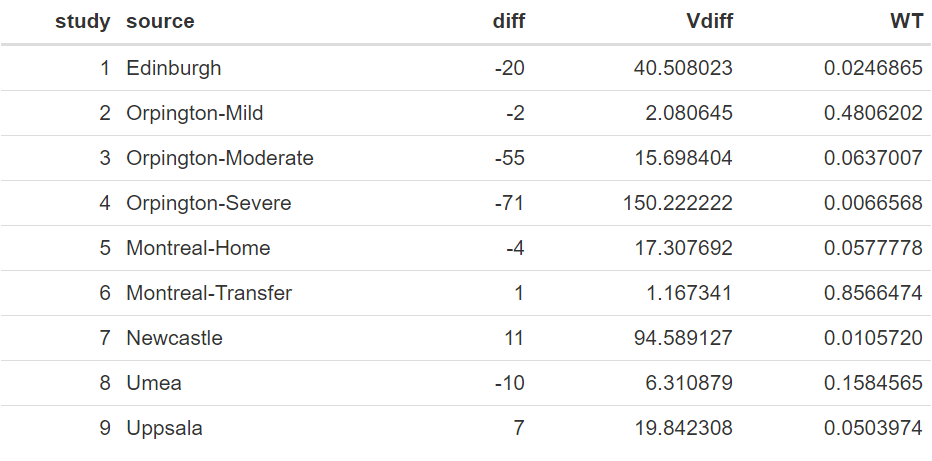The above table shows a wide range of values between the studies in the mean difference of length of stay between the two treatments, ranging from as low as −71.0 to 11.0, with a raw average of −15.9. Also, the variances of these differences vary considerably, which is also reflected in their weights.

The code to fit the fixed-effect linear model using ASReml-R is shown below:

 library(asreml) meta_f<-asreml(fixed=diff~1,                weights=WT,                family=asr_gaussian(dispersion=1),                data=STROKE)

In the above model, our response variable is diff, and the weights are indicated by the variate WT. As the precisions are contained within the weights the command family is required to fix the residual error (MSE) to exactly 1.0, hence, it will not be estimated.

The model generates output that can be used for inference. We will start by exploring our target parameter, i.e. θ, by looking at the estimated fixed effect mean and its standard error. This is done with the code:

 meta_effect <- summary(meta_f, coef=TRUE)$coef.fixed Resulting in the output: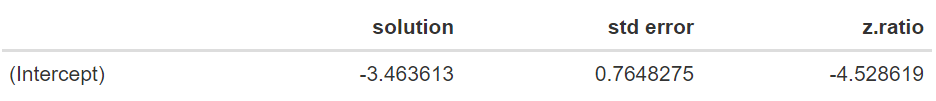The estimate of θ is equal to −3.464 days, with a standard error of 0.765. An approximate 95% confidence interval can be obtained by using a z-value of 1.96. The resulting approximate 95% confidence interval [−4.963;−1.965] does not contain zero. The significance of this value can be obtained by looking at the approximated ANOVA table using the command:  wald.asreml(meta_f) Note that this is approximated as, given that weights are considered to be known, the degrees of freedom are assumed to be infinite; hence, this will be a liberal estimate.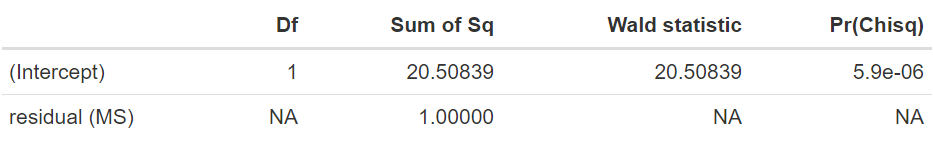The results from this ANOVA table indicate a high significance of this parameter (θ) with an approximated p-value of < 0.0001. Therefore, in summary, this fixed effect model analysis indicates a strong effect of the specialised care resulting in a reduction of approximately 3.5 days in hospitalisation. However, as indicated earlier, a random-effects model might seem more reasonable given the inherent differences in the studies under consideration. Here, we extend the model to include the random effect of study. In order to do this, first we need to ensure that this is treated as a factor in the model by running the code:  STROKE$study <- as.factor(STROKE$study)_f) The LMM to be fitted using ASReml-R is:  meta_r<-asreml(fixed=diff~1,  random=~study,  weights=WT,  family=asr_gaussian(dispersion=1),  data=STROKE) Note in this example the only difference from the previous code is the inclusion of the line random=~study. This includes the factor study as a random effect. An important result from running are the variance component estimates. These are obtained with the command:  summary(meta_r)$varcomp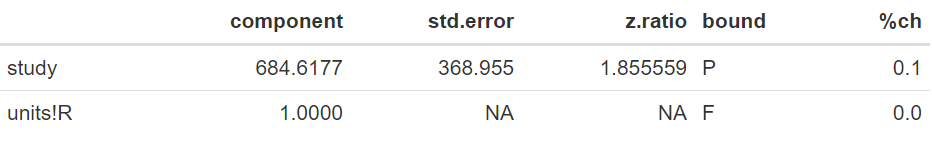In this example, the variance associated with the differences in the target parameter (θ) between the studies is 684.62. When expressed as a standard deviation, this corresponds to 26.16 days. Note that this variation is large in relation to the scale of the data, reflecting large differences between the random sample of studies considered in the meta-analysis.

We can output the fixed and random effects using the following commands:

 meta_effect <- summary(meta_r, coef=TRUE)$coef.fixed BLUP <- summary(meta_r, coef=TRUE)$coef.random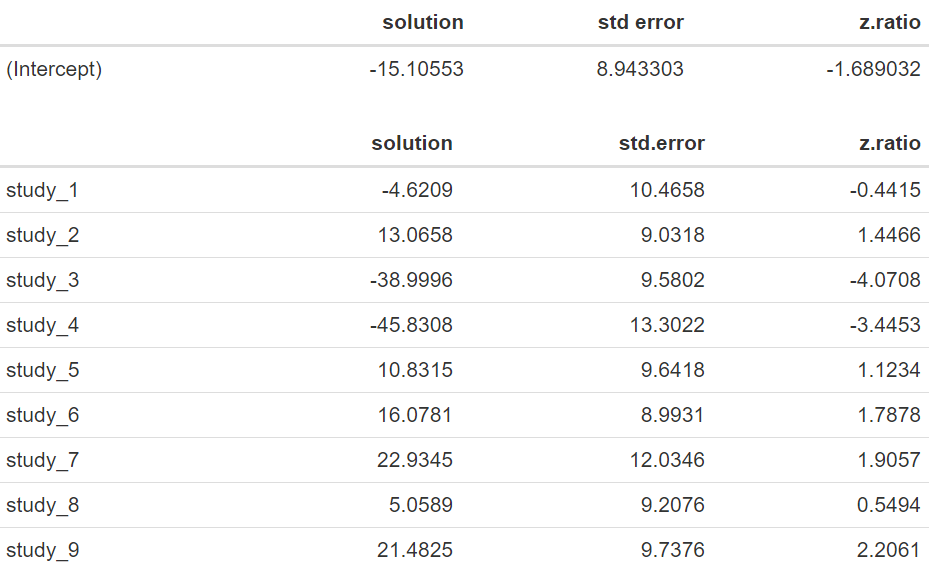Note that now that our estimated mean difference corresponds to −15.106 days with an standard error of 8.943, and that the approximate 95% confidence interval [−32.634;2.423] now contains zero. An approximated ANOVA based on the following code:

 wald.asreml(meta_r)

results in the output: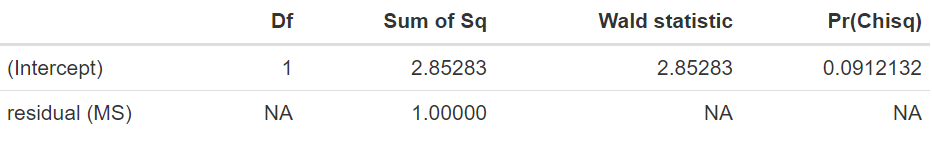We have a p-value of 0.0912, indicating that there is no significant difference in length of stay between the treatments evaluated. Note that the estimates of the random effects of study, also known as BLUPs (best linear unbiased predictions) are large, ranging from −45.8 to 22.9, and widely variable. The lack of significance in the random-effects model, when there is a difference of −15.11 days, is mostly due to the large variability of 684.62 found between the different studies, resulting in a substantial standard error for the estimated mean difference.

In the following graph we can observe the 95% confidence intervals for each of the nine studies together with the final parameter estimated under the Random-effects Model. Some of these confidence intervals contain the value zero, including the one for the random-effects model. However, it can be observed that the confidence interval from the random-effects model is an adequate summarization of the nine studies, representing a compromising confidence interval.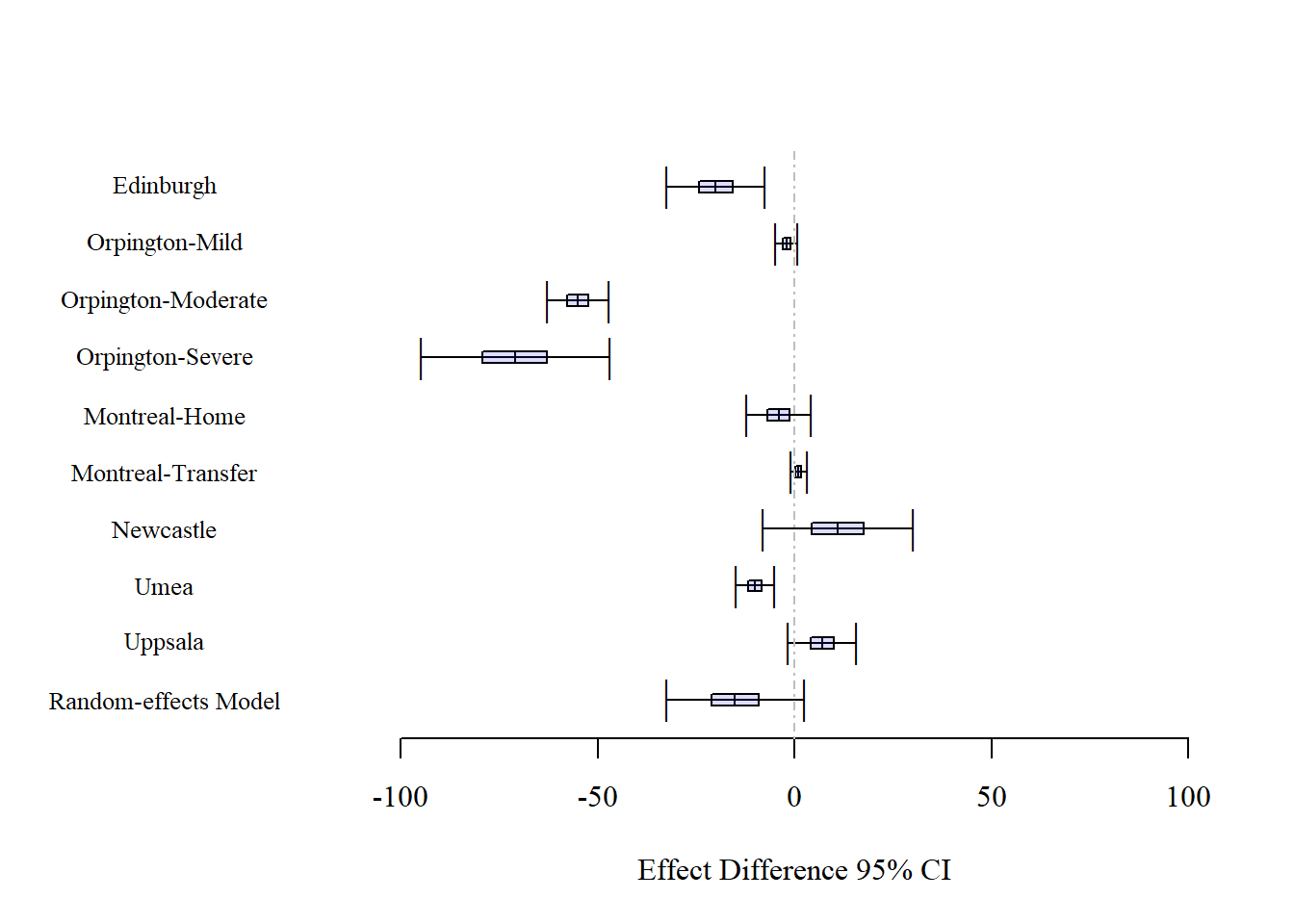An important aspect to consider is the difference in results between the fixed-effect and the random-effects model that are associated, as indicated earlier, with different inferential approaches. One way to understand this is by considering what will happen if a new random study is included. Because we have a large variability in the study effects (as denoted by ), we expect this new study to have a difference between treatments that is randomly within this wide range. This, in turn, is expressed by the large standard error of the fixed effect θ, and by its large 95% confidence interval that will ensure that for ‘any’ observation we cover the parameter estimate 95% of the time. Therefore, as shown by the data, it seems more reasonable to consider the random-effects model than the fixed-effect model as it is an inferential approach that deals with several sources of variation.

### Summary

In summary, we have used the random-effects model to perform meta-analysis on a medical research question of treatment differences by combining nine independent studies. Under this approach we assumed that all studies describe the same effect but we allowed for the model to express different effect sizes through the inclusion of a random effect that will vary from study to study. The main aim of this analysis was not to explain why these differences occur, here, our aim was to incorporate a measure of this uncertainty on the estimation of the final effect of treatment differences.

There are several extensions to meta-analysis with different types of responses and effects. Some of the relevant literature recommended to the interested reader are van Houwelingen et al. (2002) and Vesterinen et al. (2014). Also, a clear presentation with further details of the differences between fixed-effect and random-effects models is presented by Borenstein et al. (2010).

Dataset: STROKE.txt
R code: STROKE_METAA.R

### References

Borenstein, M; Hedges, LV; Higgins, JPT; Rothstein, HR. 2010. A basic introduction to fixed-effect and random-effects models for meta-analysis. Research Synthesis Methods 1: 97-111.

Butler, DG; Cullis, BR; Gilmour, AR; Gogel, BG; Thompson, R. 2017. ASReml-R Reference Manual Version 4. VSNi International Ltd, Hemel Hempstead, HP2 14TP, UK.

Normand, ST. 1999. Meta-analysis: Formulating, evaluating, combining, and reporting. Statistics in Medicine 18: 321-359.

van Houwelingen, HC; Arends, LR; Stignen, T. 2002. Advanced methods in meta-analysis: multivariate approach and meta-regression. Statistics in Medicine 21: 589-624.

Vesterinen, HM; Sena, ES; Egan, KJ; Hirst, TC; Churolov, L; Currie, GL; Antonic, A; Howells, DW; Macleod, MR. 2014. Meta-analysis of data from animal studies: a practical guide. Journal of Neuroscience Methods 221: 92-102.